You’ve just created an amazing plot in Python with Matplotlib but would like to change figure size. I will show you three different approaches to changing figure size in Matplotlib. All code samples from article can be used in Python script or Jupyter Notebook (Jupyter Lab).

Important: The figure size in Matplotlib is in inches.

In all examples, I’m using Matplotlib in version `3.6.2`.

``````# how to check your Matplotlib version
import matplotlib
print(matplotlin.__version__)

>> 3.6.2
``````

You can create an empty figure to check the default width and height in Matplotlib:

``````from matplotlib import pytplot as plt

f = plt.figure()
print(f.get_figwidth(), f.get_figheight())

>> 6.0 4.0
``````

On my system (Ubuntu 20.04, Python 3.8), the default figure size is `6.0` inches in width and `4.0` inches in height.

The figure also has a `dpi` parameter that can be adjusted with the figure size. The `dpi` is an acronym for dots per inch. It sets a number of pixels per inch. We need to multiply the figure size by `dpi` to get the figure size in pixels. On my system default `dpi` is `72.0`:

``````from matplotlib import pytplot as plt

f = plt.figure()
print(f.get_dpi())

>> 72.0
``````

Matplotlib `dpi` parameter can be used to scale fonts, line width, or marker size. It works as a magnifying glass. The figure size in Matplotlib depends on `figsize` and `dpi`.

The outline of methods to change figure size in Matplotlib:

• set `figsize` and `dpi` during figure creation,
• set size and `dpi` with setters functions: `set_figwidth`, `set_figheight`, `set_size_inches`, `set_dpi`,
• set size and `dpi` for all plots with `rcParams`.

## 1. Set `figsize` and `dpi` in the figure

The most straightforward way to set figure size is to set a `figsize` argument when creating a new figure. Below are a few examples with different figure sizes.

``````plt.figure(figsize=(3, 3))
_ = plt.plot([3, 4, 2, 5])
``````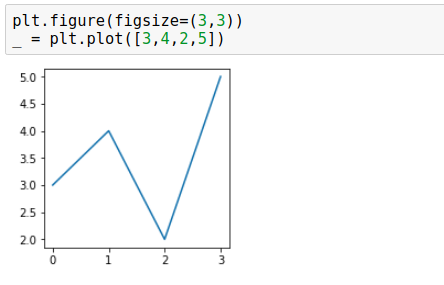``````plt.figure(figsize=(6, 3))
_ = plt.plot([3, 4, 2, 5])
``````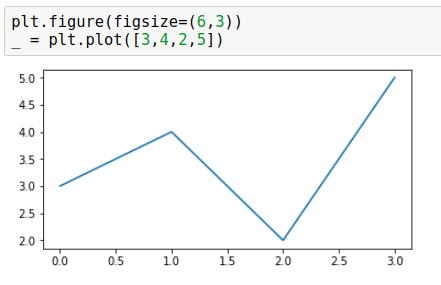``````plt.figure(figsize=(3, 6))
_ = plt.plot([3, 4, 2, 5])
``````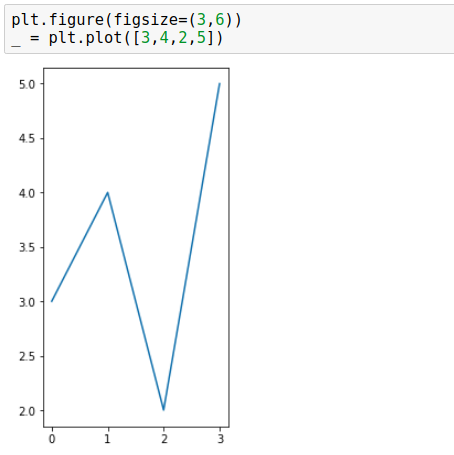The `dpi` can be passed as an argument to the `figure()` function. We can control the width of objects (axes, fonts, lines, markers) in the figure. Below are two figures, both having the same pixel size: `432x432` but different `dpi` and `figsize` values:

• `432x432` = `6x6` * `72`,
• `432x432` = `3x3` * `144`.
``````plt.figure(figsize=(6, 6), dpi=72)
_ = plt.plot([3, 4, 2, 5])
``````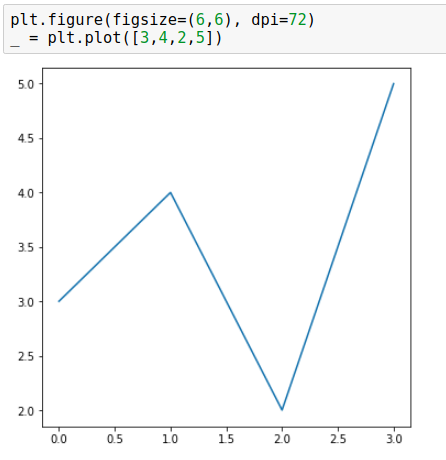``````plt.figure(figsize=(3, 3), dpi=144)
_ = plt.plot([3, 4, 2, 5])
``````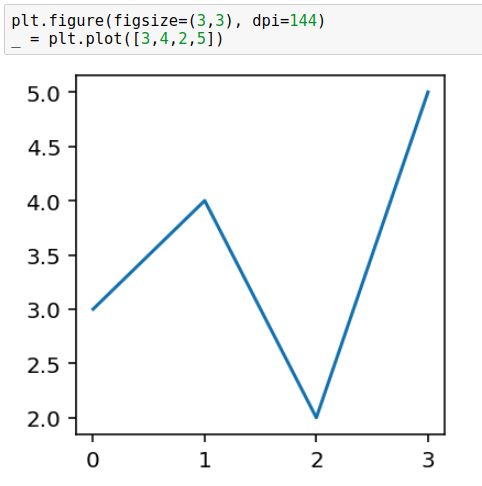As you can see in the above images, figure with a larger `dpi` has wider lines, axes, larger font, and fewer tick labels - `dpi` magnifies all elements. You can read an amazing explanation of the relationship between `dpi` and `figsize` in this StackOverflow response.

## 2. Use `set_figwidth`, `set_figheight`, `set_size_inches`, `set_dpi`

The next way to change figure size in Matplotlib is to use following functions:

• `set_figwidth` - sets figure width in inches,
• `set_figheight` - sets figure height in inches,
• `set_size_inches` - set figure width and height in inches,
• `set_dpi` - sets figure `dpi`.

Below are two examples that change figure size and set `dpi`.

``````# create figure
f = plt.figure()

# set width, height, dpi
f.set_figwidth(4)
f.set_figheight(2)
f.set_dpi(142)

# plot
_ = plt.plot([3,4,2,5])
``````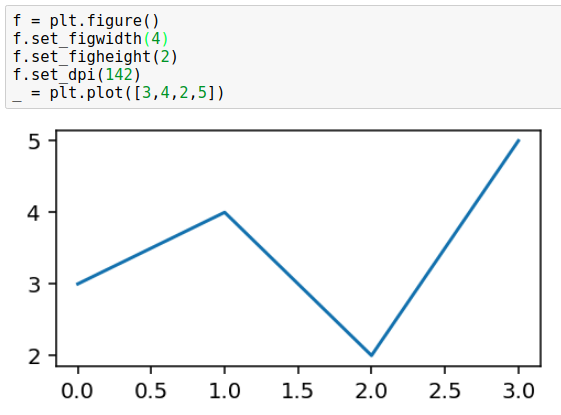Change figure size with `set_size_inches(w, h)`:

``````# create figure
f = plt.figure()

# set size, dpi
f.set_size_inches(4, 2)
f.set_dpi(142)

# plot
_ = plt.plot([3,4,2,5])
``````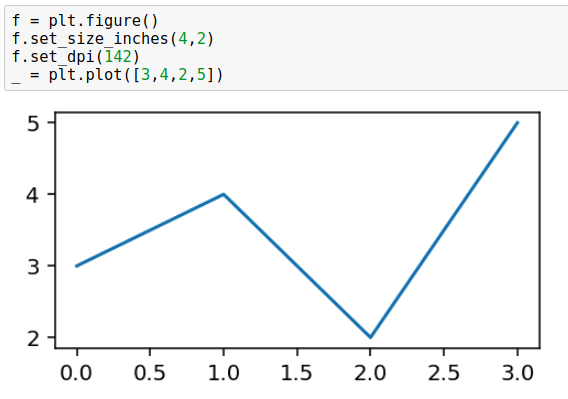## 3. Change figure size with `rcParams`

The last option is to set figure size globally to all figures plotted in the script or notebook. We use this `rcParams`.

``````# set figsize and dpi for all figures
plt.rcParams["figure.figsize"] = (4,2)
plt.rcParams["figure.dpi"] = 144
``````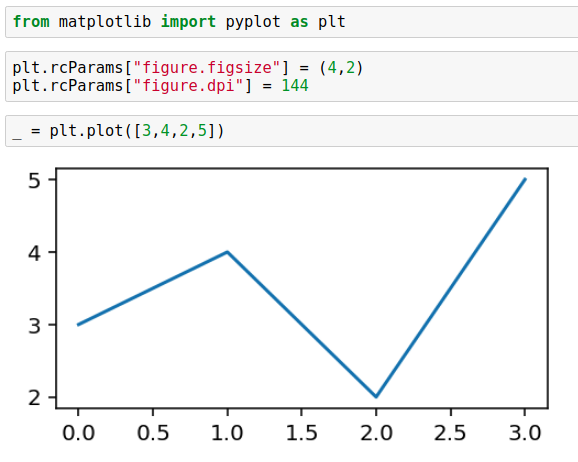This option sets figure size and dpi for all figures in the current script or notebook.

For Jupyter Notebook users: please set `rcParams` in a separate cell below imports. Setting `rcParams` in the same cell as `matplotlib` import will not make any change.

## Summary

Matplotlib is a popular plotting library for Python. You need to correctly understand the relationship between figure size and `dpi` to get the desired size of the plot. You can set figure size and `dpi` when creating a new figure. If you already have a figure created (or it was created by another package) then you can manipulate its size and `dpi` with `set_figwidth`, `set_figheight`, `set_size_inches`, `set_dpi` functions. There is an option to set figure size and `dpi` globally to all figures in the script (or notebook) by using `rcParams`.### Convert Python Notebooks to Web Apps

We are working on open-source framework Mercury for converting Jupyter Notebooks to interactive Web Applications.### Articles you might find interesing